# 为什么阿里巴巴禁止使用BigDecimal的equals方法做等值比较？

Hollis的新书《深入理解Java核心技术》出版了

BigDecimal，相信对于很多人来说都不陌生，很多人都知道他的用法，这是一种java.math包中提供的一种可以用来进行精确运算的类型。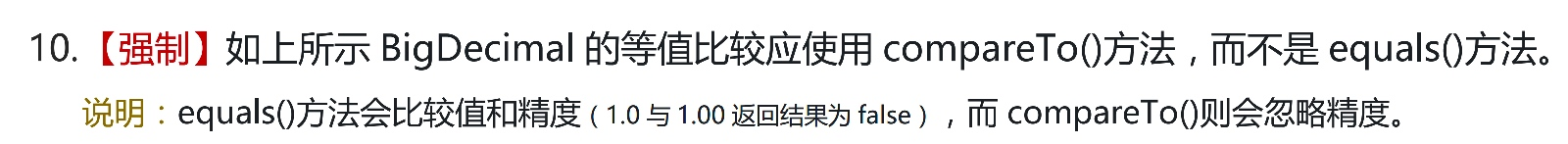``````if(bigDecimal == bigDecimal1){
// 两个数相等
}
``````

``````if(bigDecimal.equals(bigDecimal1)){
// 两个数相等
}
``````

``````BigDecimal bigDecimal = new BigDecimal(1);
BigDecimal bigDecimal1 = new BigDecimal(1);
System.out.println(bigDecimal.equals(bigDecimal1));

BigDecimal bigDecimal2 = new BigDecimal(1);
BigDecimal bigDecimal3 = new BigDecimal(1.0);
System.out.println(bigDecimal2.equals(bigDecimal3));

BigDecimal bigDecimal4 = new BigDecimal("1");
BigDecimal bigDecimal5 = new BigDecimal("1.0");
System.out.println(bigDecimal4.equals(bigDecimal5));
``````

``````true
true
false
``````

### BigDecimal的equals原理

``````Compares this  BigDecimal with the specified Object for equality.  Unlike compareTo, this method considers two BigDecimal objects equal only if they are equal in value and scale (thus 2.0 is not equal to 2.00 when compared by  this method)
``````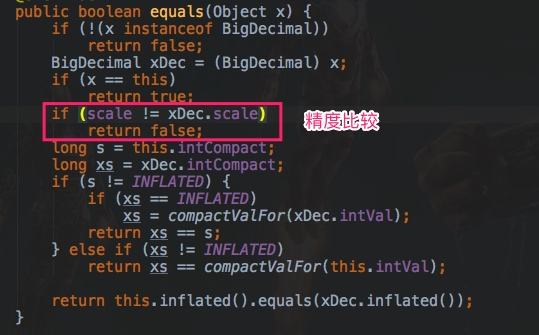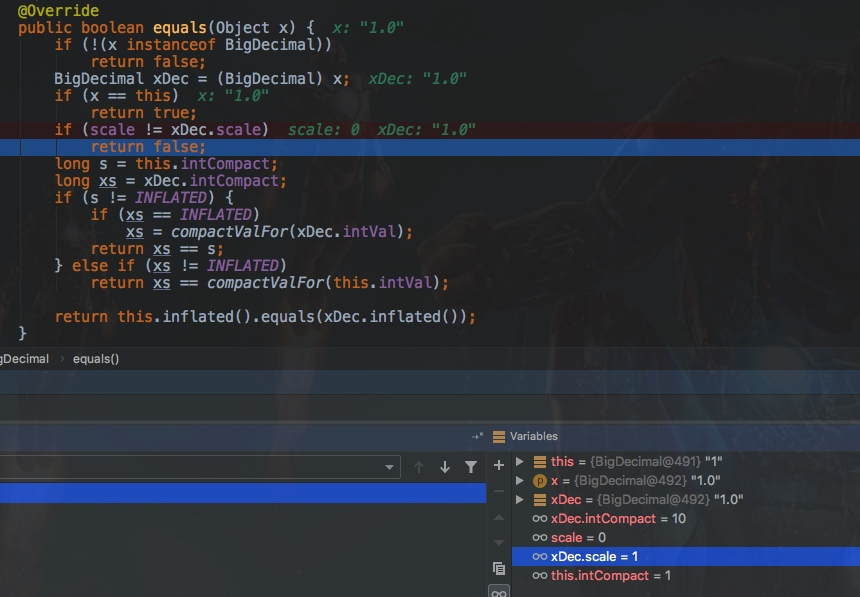### 为什么标度不同

``````BigDecimal(int)
BigDecimal(double)
BigDecimal(long)
BigDecimal(String)
``````

#### BigDecimal(long) 和BigDecimal(int)

``````public BigDecimal(int val) {
this.intCompact = val;
this.scale = 0;
this.intVal = null;
}

public BigDecimal(long val) {
this.intCompact = val;
this.intVal = (val == INFLATED) ? INFLATED_BIGINT : null;
this.scale = 0;
}
``````

#### BigDecimal(double)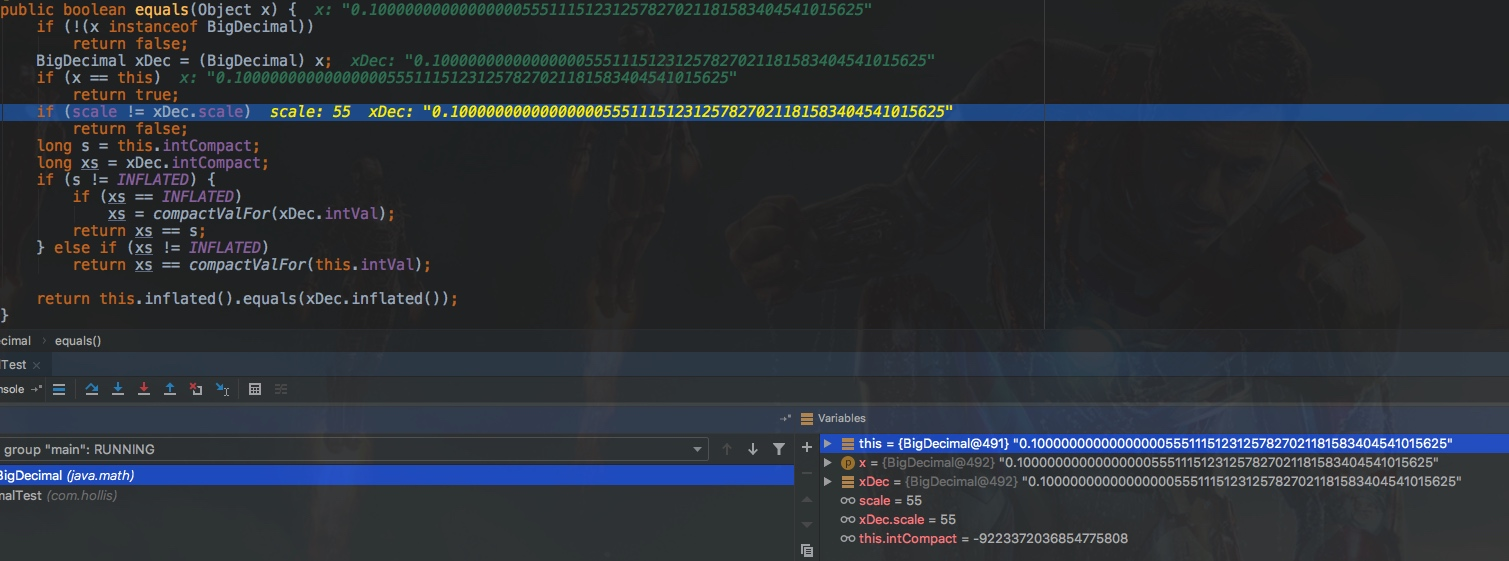### 如何比较BigDecimal

BigDecimal中提供了compareTo方法，这个方法就可以只比较两个数字的值，如果两个数相等，则返回0。

``````    BigDecimal bigDecimal4 = new BigDecimal("1");
BigDecimal bigDecimal5 = new BigDecimal("1.0000");
System.out.println(bigDecimal4.compareTo(bigDecimal5));
``````

``````0
``````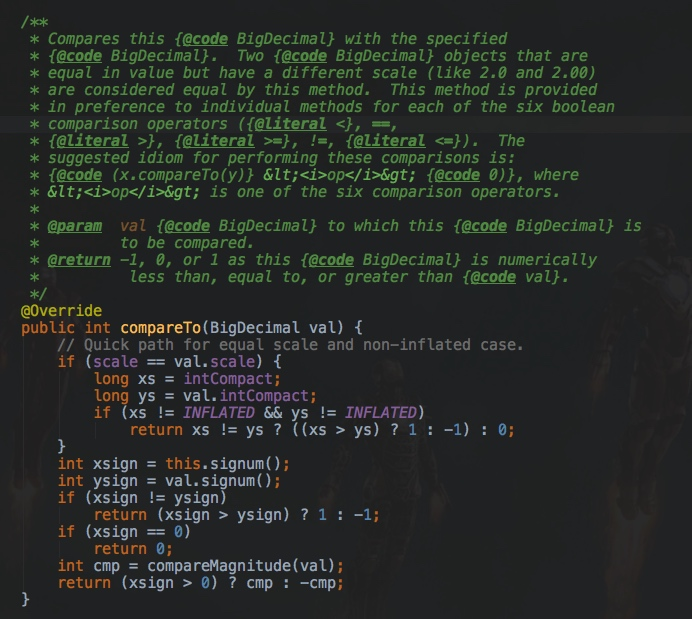### 总结

BigDecimal是一个非常好用的表示高精度数字的类，其中提供了很多丰富的方法。(全文完)### 评论 1

1. #1

可以出一版，所有类型的比较方式，integer,int等等，什么用==，什么时候用equal

w.fantasy2年前 (2020-09-21)回复

## HollisChuang's Blog

• 微信咨询
• 去评论
• 回顶
###### 回顶部